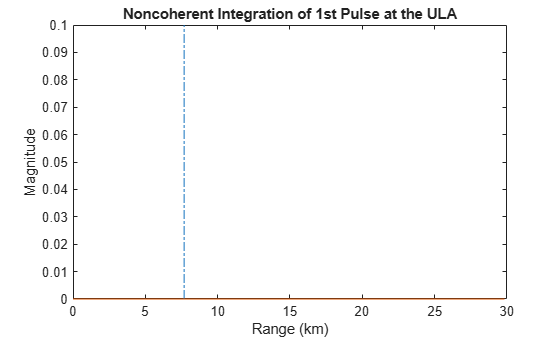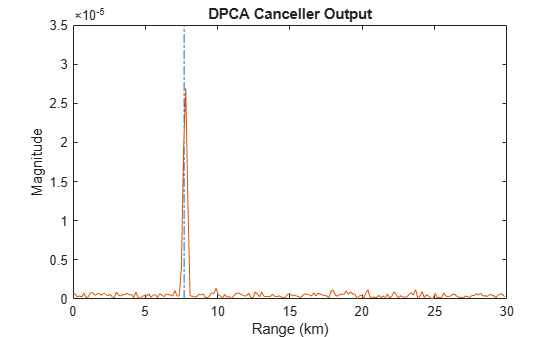## Displaced Phase Center Antenna Pulse Canceller

### When to Use the DPCA Pulse Canceller

In a moving target indication (MTI) radar, clutter returns can make it more difficult to detect and track the targets of interest. A rudimentary way to mitigate the effects of clutter returns in such a system is to implement a displaced phase center antenna (DPCA) pulse canceller on the slow-time data.

You can implement a DPCA pulse canceller with `phased.DPCACanceller`. This implementation assumes that the entire array is used on transmit. On receive, the array is divided into two subarrays. The phase centers of the subarrays are separated by twice the distance the platform moves in one pulse repetition interval.

#### Applicability of DPCA Pulse Canceller

The DPCA pulse canceller is applicable when both these conditions are true:

• Clutter is stationary across pulses.

• The motion satisfies

 $vT=d/2$ (1)

where:

• v indicates the speed of the platform

• T represents the pulse repetition interval

• d indicates the interelement spacing of the array

### DPCA Pulse Canceller to Reject Clutter

This example implements a DPCA pulse canceller for clutter rejection. Assume you have an airborne radar platform modeled by a six-element ULA operating at 4 GHz. The array elements are spaced at one-half the wavelength of the 4 GHz carrier frequency. The radar emits ten rectangular pulses two microseconds in duration with a PRF of 5 kHz. The platform moves along the array axis with a speed equal to one-half the product of the element spacing and the PRF. DPCA pulse cancellation is applicable because $vT=d/2$ where

• $v$ indicates the speed of the platform

• $T$ represents the pulse repetition interval

• $d$ indicates the interelement spacing of the array

The target has a nonfluctuating RCS of 1 square meter and moves with a constant velocity vector of (15,15,0).

```PRF = 5e3; fc = 4e9; fs = 1e6; c = physconst('LightSpeed'); antenna = phased.IsotropicAntennaElement... ('FrequencyRange',[8e8 5e9],'BackBaffled',true); lambda = c/fc; array = phased.ULA(6,'Element',antenna,'ElementSpacing',lambda/2); waveform = phased.RectangularWaveform('PulseWidth',2e-6,... 'PRF',PRF,'SampleRate',fs,'NumPulses',1); radiator = phased.Radiator('Sensor',array,... 'PropagationSpeed',c,... 'OperatingFrequency',fc); collector = phased.Collector('Sensor',array,... 'PropagationSpeed',c,... 'OperatingFrequency',fc); vy = (array.ElementSpacing*PRF)/2; transmitplatform = phased.Platform('InitialPosition',[0;0;3e3],... 'Velocity',[0;vy;0]); target = phased.RadarTarget('MeanRCS',1,... 'Model','Nonfluctuating','OperatingFrequency',fc); targetplatform = phased.Platform('InitialPosition',[5e3; 5e3; 0],... 'Velocity',[15;15;0]); channel = phased.FreeSpace('OperatingFrequency',fc,... 'TwoWayPropagation',false,'SampleRate',fs); receiver = phased.ReceiverPreamp('NoiseFigure',0,... 'EnableInputPort',true,'SampleRate',fs,'Gain',40); transmitter = phased.Transmitter('PeakPower',1e4,... 'InUseOutputPort',true,'Gain',40); % Load simulated clutter data load clutterdata```

Propagate ten rectangular pulses to target and back, and collect the responses at the array. Also, compute clutter echoes using the constant gamma model with a gamma value corresponding to wooded terrain.

```NumPulses = 10; wav = waveform(); M = fs/PRF; N = array.NumElements; rxsig = zeros(M,N,NumPulses); csig = zeros(M,N,NumPulses); fasttime = unigrid(0,1/fs,1/PRF,'[)'); rangebins = (c*fasttime)/2; for n = 1:NumPulses [txloc,txvel] = transmitplatform(1/PRF); % move transmitter [tgtloc,tgtvel] = targetplatform(1/PRF); % move target [~,tgtang] = rangeangle(tgtloc,txloc); % get angle to target [txsig1,txstatus] = transmitter(wav); % transmit pulse txsig = radiator(txsig1,tgtang); % radiate pulse txsig = channel(txsig,txloc,tgtloc,... txvel,tgtvel); % propagate to target txsig = target(txsig); % reflect off target txsig = channel(txsig,tgtloc,txloc,... tgtvel,txvel); % propagate to array rxsig(:,:,n) = collector(txsig,tgtang); % collect pulse rxsig(:,:,n) = receiver(rxsig(:,:,n) + csig(:,:,n),... ~txstatus); % receive pulse plus clutter return end```

Determine the target range, range gate, and two-way Doppler shift.

```sp = radialspeed(tgtloc,tgtvel,txloc,txvel); tgtdoppler = 2*speed2dop(sp,lambda); tgtLocation = global2localcoord(tgtloc,'rs',txloc); tgtazang = tgtLocation(1); tgtelang = tgtLocation(2); tgtrng = tgtLocation(3); tgtcell = val2ind(tgtrng,c/(2*fs));```

Use noncoherent pulse integration to visualize the signal received by the ULA for the first of the ten pulses. Mark the target range gate with a vertical dashed line.

```firstpulse = pulsint(rxsig(:,:,1),'noncoherent'); plot([tgtrng/1e3 tgtrng/1e3],[0 0.1],'-.',rangebins/1e3,firstpulse) title('Noncoherent Integration of 1st Pulse at the ULA') xlabel('Range (km)') ylabel('Magnitude');```The large-magnitude clutter returns obscure the presence of the target. Apply the DPCA pulse canceller to reject the clutter.

```canceller = phased.DPCACanceller('SensorArray',array,'PRF',PRF,... 'PropagationSpeed',c,... 'OperatingFrequency',fc,... 'Direction',[0;0],'Doppler',tgtdoppler,... 'WeightsOutputPort',true); [y,w] = canceller(rxsig,tgtcell);```

Plot the result of applying the DPCA pulse canceller. Mark the target range gate with a vertical dashed line.

```plot([tgtrng/1e3,tgtrng/1e3],[0 3.5e-5],'-.',rangebins/1e3,abs(y)) title('DPCA Canceller Output') xlabel('Range (km)') ylabel('Magnitude')```The DPCA pulse canceller has significantly rejected the clutter. As a result, the target is visible at the expected range gate.